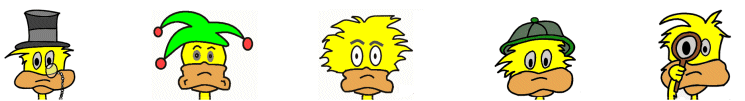# Kids Math

## Introduction to Fractions

What is a fraction?

A fraction represents part of a whole. When something is broken up into a number of parts, the fraction shows how many of those parts you have.

Pictures of Fractions

Sometimes the best way to learn about fractions is through a picture. See the pictures below to see how the whole of a circle can be broken up into different fractions. The first picture shows the whole and then the other pictures show fractions of that whole.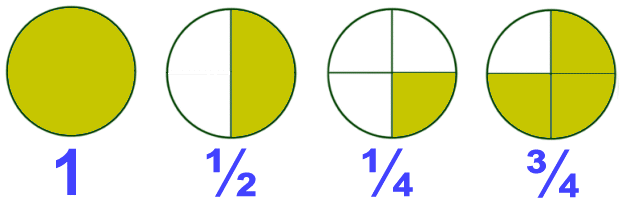Numerator and Denominator

When writing a fraction there are two main parts: the numerator and the denominator. The numerator is how many parts you have. The denominator is how many parts the whole was divided into.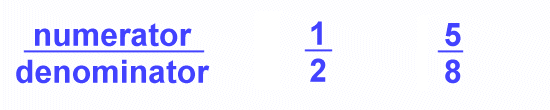Fractions are written with the numerator over the denominator and a line in between them.

Types of Fractions

There are three different types of fractions:

1. Proper Fractions - A proper fraction is one where the numerator is less than the denominator. Note that a proper fraction is always less than one.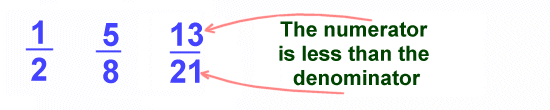2. Improper Fractions - An improper fraction is one where the numerator is greater than the denominator. Note that an improper fraction is always greater than one.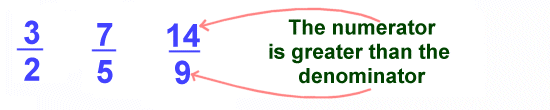3. Mixed Fractions - A mixed fraction had both a whole number part and a fractional part.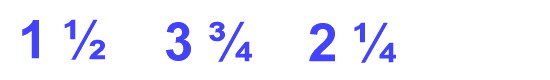Reciprocals

A reciprocal is a fraction where the numerator and denominator are reversed. It can also be looked at as 1 over the number. When you take a number or fraction and multiply it by its reciprocal, the answer is always 1.

Equivalent Fractions

Sometimes fractions may look different and have different numbers, but they are equivalent or have the same value.

One of the simplest examples of equivalent fractions is the number 1. If the numerator and the denominator are the same, then the fraction has the same equivalent value as 1.Here are some equivalent fractions for 3/4. The equivalent fractions are all multiples of 3/4. Take 15/20 for example. 3x5 = 15 and 4x5 = 20.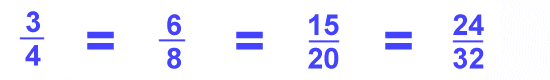Go here for more on equivalent fractions.

Decimals

When decimal points are used in numbers, the number to the right of the decimal point is a type of fraction. Depending on the place value it can be 1/10, 1/100, 1/1000 or some other factor of 10.

Examples:

0.3 = 3/10
0.42 = 42/100

Percents

Another type of fraction is the percent. The "percent" is a fraction with a denominator of 100. When you say 50% it is the same as saying 50/100.

Back to Kids Math

Back to Kids Study